• 在BERT（pre-training of deep bidirectional transformers）预训练语言模型的基础上，提出一种基于残差空洞卷积神经网络和条件随机场的网络安全实体识别模型 BERT-RDCNN-CRF。通过BERT模型训练字符级特征向量表示，...网络安全 实体识别 BERT预训练模型
• 博客见：https://blog.csdn.net/qq_37534947/article/details/109727232，主要是空洞卷积以及残差网络的代码实现，包含数据集，框架是pytorch
• 基于残差空洞卷积神经网络的网络安全实体识别方法.pdf
• 二维卷积实验 卷积神经网络是一种具有局部连接、权重共享特性的深层前馈神经网络。局部连接是指通过引入卷积核，使得每次运算学习的特征只和局部输入相关，极大地减少了计算量和连接层数；权值共享是指学习的特征...
Pytorch系列文章：

Pytorch实验一：从零实现Logistic回归和Softmax回归Pytorch实验2：手动实现前馈神经网络、Dropout、正则化、K折交叉验证，解决多分类、二分类、回归任务Pytorch实验3：动手实现卷积神经网络、空洞卷积、残差网络Pytorch实验4：手动/torch实现三种循环神经网络解决交通流量预测任务Pytorch实验5：疫情微博文本情感分类 (简化版SMP2020赛题)Pytorch实验6：论文代码中的高级语法

算法系列文章

算法1：遍历与枚举算法2：分而治之

大数据系列文章

目录：实现卷积神经网络、空洞卷积、残差网络
实验内容二维卷积实验空洞卷积实验残差网络实验
实验环境及实验数据集实验过程二维卷积实验手动实现二维卷积使用torch实现二维卷积对不同超参数的实验结果对比分析复现AlexNet模型与前馈神经网络的效果进行对比
空洞卷积实验残差网络实验
实验结果二维卷积实验手动实现二维卷积使用torch实现二维卷积对不同超参数的实验结果对比分析卷积核大小的影响卷积层数的影响
复现AlexNet模型与前馈神经网络的效果进行对比
空洞卷积实验空洞卷积实验结果空洞卷积与普通卷积的效果对比超参数的对比卷积核大小的影响卷积层数的影响

残差网络实验残差网络实验结果残差空洞卷积实验结果

实验心得体会参考文献写文不易 欢迎投喂！

实验内容
二维卷积实验
卷积神经网络是一种具有局部连接、权重共享特性的深层前馈神经网络。局部连接是指通过引入卷积核，使得每次运算学习的特征只和局部输入相关，极大地减少了计算量和连接层数；权值共享是指学习的特征具有局部不变性，使得对此类特征提取更加充分。 在深度学习中，卷积的定义和分析数学、信号处理中的定义略有不同。一般地，卷积通过互相关(cross-correlation)计算。给定输入

X

∈

R

M

×

N

\textbf{X}\in R^{M\times N}

和卷积核

W

∈

R

U

×

V

\textbf{W}\in R^{U\times V}

，它们的互相关为

y

i

,

j

=

∑

u

=

1

U

∑

v

=

1

V

w

u

,

v

x

i

+

u

−

1

,

j

+

v

+

1

y_{i,j}= \sum_{u=1}^{U}{ \sum_{v=1}^{V}{w_{u,v}x_{i+u-1,j+v+1}}}

也记作：

Y

=

W

⊗

X

\textbf{Y}=\textbf{W}⊗\textbf{X}

F

F

)、卷积核大小(

K

K

)、步长(

S

S

)、填充(

P

P

)，和输入图片的通道数(

D

D

)、宽度(

W

W

)、高度(

H

H

)共同决定；其中输出的通道数、宽度、高度分别是：

D

o

u

t

=

F

D_{out}=F

W

o

u

t

=

W

+

2

P

−

K

S

+

1

W_{out}=\frac{W+2P-K}{S}+1

H

o

u

t

=

H

+

2

P

−

K

S

+

1

H_{out}=\frac{H+2P-K}{S}+1

对于每个卷积核，共引入

K

×

K

×

D

+

1

K\times K\ \times D\ +1

个参数；由于有F个卷积核，所以共有

F

×

D

×

K

2

+

F

F\times D\times K^2+F

个参数。 卷积层(Convolution Layer)通常与汇聚层(Pooling Layer)混合使用。汇聚层是一种下采样(down sample)操作，作用是减少参数和特征的数量、加快运算速度和增加模型的鲁棒性。常用的汇聚包括：最大汇聚和平均汇聚。 二维卷积实验的目的是：（1）手动实现二维卷积；（2）使用torch实现二维卷积；（3）进行实验结果的分析、超参数的对比分析；（4）复现AlexNet模型；（5）与前馈神经网络的效果进行对比。
空洞卷积实验
空洞卷积是指通过在卷积核的元素间插入空隙来增加感受野的方法。对于大小为

K

×

K

K\times K

的卷积核，在每两个元素中插入

D

−

1

D-1

个元素，则空洞卷积核的有效大小是：

K

′

=

K

+

(

K

−

1

)

×

(

D

−

1

)

K'=K+(K-1)×(D-1)

其中：D是空洞卷积的膨胀率。例如：原卷积核大小为

3

×

3

3\times3

，则空洞卷积核的单边大小为

3

+

(

3

−

1

)

×

(

2

−

1

)

=

5

3+\left(3-1\right)\times\left(2-1\right)=5

。普通卷积可以视为

D

=

1

D=1

时的空洞卷积。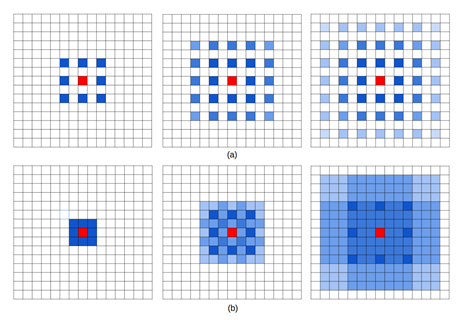由于空洞卷积引入了空隙，有一部分特征没有参与计算，且捕获具有一定间隔的特征可能是不相关的。Panqu Wang等人在2018年提出混合空洞卷积条件（Hybrid Dilated Convolution, HDC），对网络中每一层的膨胀率变化规律做出规定。满足此条件的网络可以规避特征空隙、同时捕获远程和近距离信息。 对于卷积核大小均为

K

×

K

K\times K

的N层卷积神经网络，其每一层膨胀率组成的序列分别为：

[

r

1

,

…

,

r

i

,

…

,

r

n

]

\left[r_1,\ldots,r_i,\ldots,r_n\right]

，需满足:

∀

1

<

i

<

n

,

M

i

≤

K

∀1<i<n,M_i≤K

其中：

M

i

=

m

a

x

[

r

i

+

1

−

2

r

i

,

2

r

i

−

r

i

+

1

,

r

i

]

Mi=max[r_{i+1}-2r_i,2r_i-r_{i+1},r_i]

本实验的目的是：（1）利用torch.nn实现空洞卷积，其中：膨胀率序列(dilation)满足HDC条件，在车辆分类数据集上实验并输出结果；（2）比较空洞卷积和普通卷积的结果；（3）选取1-2个超参数进行对比分析。
残差网络实验
在深度神经网络中，如果期望非线性单元

f

(

x

;

θ

)

f\left(\textbf{x};\theta\right)

去逼近目标函数

h

(

x

)

h(\textbf{x})

；可以考虑将目标函数分解为：

h

(

x

)

=

x

+

h

(

x

)

−

x

h(\textbf{x})=\textbf{x}+h(\textbf{x})-\textbf{x}

其中，

x

\textbf{x}

称为恒等函数(identity function)，

(

h

(

x

)

−

x

)

(h(\textbf{x})-\textbf{x})

称为残差函数(residue function)。 根据通用近似定理，非线性单元可以在理论上逼近任意函数。因此，原问题可以进行如下转化：期望

f

(

x

)

f\left(\textbf{x}\right)

逼近残差函数

(

h

(

x

)

−

x

)

(h(\textbf{x})-\textbf{x})

，使得

f

(

x

)

+

x

f\left(\textbf{x}\right)+\textbf{x}

逼近目标函数

h

(

x

)

h(\textbf{x})

。 一般地，残差网络可以通过跳层连接(shortcut connection)实现。过往的实验表明：残差网络可以解决深层神经网络的梯度爆炸、梯度消失和网络退化问题。 本实验的目的是：（1）复现给定的残差网络架构，分析结果；（2）结合空洞卷积，对比分析实验结果。
实验环境及实验数据集
数据集采用车辆分类数据集，共1358张车辆图片，三个类别。其中：随机抽取70%作训练集，30%作测试集。 实验环境为Linux 3.10.0-1062.el7.x86_64；运算器为NVIDIA GeForce RTX 2080；框架为：Pytorch 1.6.0；采用Pycharm内置的SSH连接进行交互。
实验过程
二维卷积实验
手动实现二维卷积
要实现卷积，需要依次实现：单通道互相关运算、多通道互相关运算、多卷积核互相关运算。需要说明的是，在Open CV读入的图片格式中，通道(channel)是最后一个维度，因此需要对读取的数据进行通道变换。代码段分别如下。
#单通道互相关运算
def corr2d(X,K):
batch_size,H,W = X.shape
h,w = K.shape,K.shape
Y = torch.zeros(batch_size,H-h+1,W-w+1).to(device)
for i in range(Y.shape):
for j in range(Y.shape):
area = X[:,i:i+h, j:j+w]
Y[:,i,j] = (area* K).sum()
return Y

#多通道互相关运算
def corr2d_multi_in(X, K):
res = corr2d(X[:,:,:,0],K[:,:,0])
for i in range(0,X.shape):
res += corr2d(X[:,:,:,i],K[:,:,i])
return res

#多卷积核互相关运算
def corr2d_multi_in_out(X, K):

定义了互相关运算、二维池化运算后，我们可以把卷积封装成卷积层模块。
#定义池化层
def pool2d(X, pool_size, mode='max'):
p_h, p_w = pool_size,pool_size
Y = torch.zeros((X.shape,X.shape,X.shape - p_h + 1, X.shape - p_w + 1))
for i in range(Y.shape):
for j in range(Y.shape):
if mode == 'max':
Y[:,:,i, j] = X[:,:,i: i + p_h, j: j + p_w].max()
elif mode == 'avg':
Y[:,:,i, j] = X[:,:,i: i + p_h, j: j + p_w].mean()
return Y

#定义卷积模块
class MyConv2D(nn.Module):
def __init__(self,in_channels,out_channels,kernel_size):
super(MyConv2D,self).__init__()
if isinstance(kernel_size,int):
kernel_size = (kernel_size,kernel_size)
self.weight = nn.Parameter(torch.randn((out_channels,in_channels)+kernel_size))
self.bias = nn.Parameter(torch.randn(out_channels,1,1))
def forward(self,x):
y = corr2d_multi_in_out(x,self.weight) +self.bias
return y


使用torch实现二维卷积
使用Pytorch可以直接定义模型完成训练。值得注意的是，由于卷积层的参数和输入图片大小会影响全连接层的参数设置，每一次调整网络架构时，全连接层的维度都需要重新计算。 此次实验中，我们把数据集中的图片压缩为200*100的尺寸，根据公式(3)~(5)，我们可以得出全连接层的维度是15532.
class ConvModule(nn.Module):
def __init__(self):
super(ConvModule,self).__init__()
self.conv = nn.Sequential(
nn.BatchNorm2d(32),
nn.ReLU(inplace=True)
)
self.pool = nn.MaxPool2d(2,2)
self.fc = nn.Linear(155232, num_classes)
def forward(self,X):
out = self.conv(X.float())
out = self.pool(out)
out = out.view(out.size(0), -1) # flatten each instance
out = self.fc(out)
return out

对不同超参数的实验结果对比分析
此部分，我们试验了不同的卷积层层数（在ConvModule中定义）和卷积核大小。
复现AlexNet模型
AlexNet中引入了Dropout、ReLU激活和局部相应归一化技巧，以提升模型的泛化能力。AlexNet中的局部响应归一化(Local Response Normalization, LRN)是一种对于同层的部分神经元的归一化方法。它受到生物学中侧抑制现象的启发，即：活跃神经元对邻近神经元由平衡和约束作用。数学上，可视作在激活函数的基础上，再做一次简单线性回归。此方法现已被池化层取代，很少应用。此处，我们直接采用中的LRN实现.
AlexNet是第一个现代深度网络模型，由Krizhevsky等人在2012年参与ImageNet竞赛中提出。由于当时GPU的运算性能限制，当时被拆分为两个子网络进行分别训练。它包含5个卷积层、3个汇聚层和3个全连接层。 在本实验中，我们主要参照中的AlexNet类进行实验。需要注意的是，多层网络、LRN、ReLU激活可能会带来大规模的梯度爆炸问题，造成损失为NaN。因此，我们在每一层卷积的末尾额外添加了一个BatchNorm层进行归一化处理。
与前馈神经网络的效果进行对比
我们将卷积网络与实验2中的网络（代码如下）进行对比。
layers = collections.OrderedDict([
('L1',nn.Linear(154587,192)),
('A1',nn.Hardswish()),
('drop1', nn.Dropout(p=0.1)),
('L2', nn.Linear(192, 96)),
('A2', nn.LeakyReLU()),
('drop2', nn.Dropout(p=0.1)),
('FC', nn.Linear(96,3))
])
AnnNet = nn.Sequential(layers)


空洞卷积实验
在torch中，定义空洞卷积较为简便，只需要修改卷积层的dilation参数即可。
nn.Conv2d(in_channels=3, out_channels=32, kernel_size=3, stride=1, padding=0, dilation=3) #膨胀率为3

残差网络实验由于复现的残差网络具有一定的子结构相似性，此处，我们进行了模块化处理。首先，我们定义两层卷积的残差网络：
class Residual(nn.Module):
def __init__(self, input_channels,num_channels, use_1x1conv=False, strides=1, **kwargs):
super(Residual,self).__init__()
if use_1x1conv:
self.conv3 = nn.Conv2d(num_channels,num_channels, kernel_size=1,stride=strides)
else:
self.conv3 = None
# AUTHOR:ZIXIN QIN
self.bn1 = nn.BatchNorm2d(num_channels)
self.bn2 = nn.BatchNorm2d(num_channels)

def forward(self, X):
Y = self.bn1(self.conv1(X))
Y = F.relu(Y)
Y = self.bn2(self.conv2(Y))
if self.conv3:
X = self.conv3(X)
return F.relu(Y + X)
return F.relu(Y)


之后，我们定义一层上采样、一层卷积的残差网络：
class UpsampleResidual(nn.Module):
def __init__(self, in_channel,out_channel, kernel_size=3,strides=1, **kwargs):
super(UpsampleResidual, self).__init__()
self.l1 = nn.Conv2d(in_channels=in_channel, out_channels=out_channel, kernel_size=kernel_size, stride=strides,padding=1)
self.shortcut = nn.Conv2d(in_channels=in_channel,out_channels=out_channel,kernel_size=1,stride=strides)
def forward(self,X):
Y = self.l1(X)
Y = F.relu(Y)
Y = self.l2(Y)
shortcut = self.shortcut(X)
return F.relu(Y+ shortcut)


最终，我们将以上两个模块进行多次复合，得到需要复现的网络：
class ResidualModule(nn.Module):
def __init__(self):
super(ResidualModule,self).__init__()
self.conv1 = nn.Conv2d(3,64,3)
self.conv2 = Residual(64,64,True)
self.conv3 = UpsampleResidual(64,128,3)
self.conv4 = Residual(128,128, True)
self.conv5 = UpsampleResidual(128,256,3)
self.conv6 = Residual(256,256, True)
self.conv7 = UpsampleResidual(256,512,3)
self.conv8 = Residual(512,512,True)
self.pool = torch.nn.AvgPool2d(kernel_size=3)
self.fc = nn.Linear(460800, num_classes)
self.process = nn.Sequential(self.conv1,self.conv2,self.conv3,
self.conv4,self.conv5,self.conv6,self.conv7,self.conv8)
def forward(self,X):
out = self.process(X)
out = out.view(out.size(0), -1) # flatten each instance
out = self.fc(out)
return out

实验结果
二维卷积实验
手动实现二维卷积
手动定义的卷积没有进行矩阵优化，时间复杂度为

O

(

n

4

)

\mathcal{O}\left(n^4\right)

，对运算时间要求较高。经过手动验证，反向传播过程耗费时间过长，无法预计完成模型训练的时间；因此，这里不能提供具体的手动实现的卷积的结果图表，但是可以观察到loss的计算过程。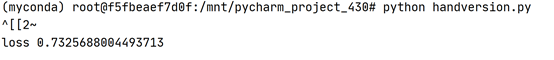使用torch实现二维卷积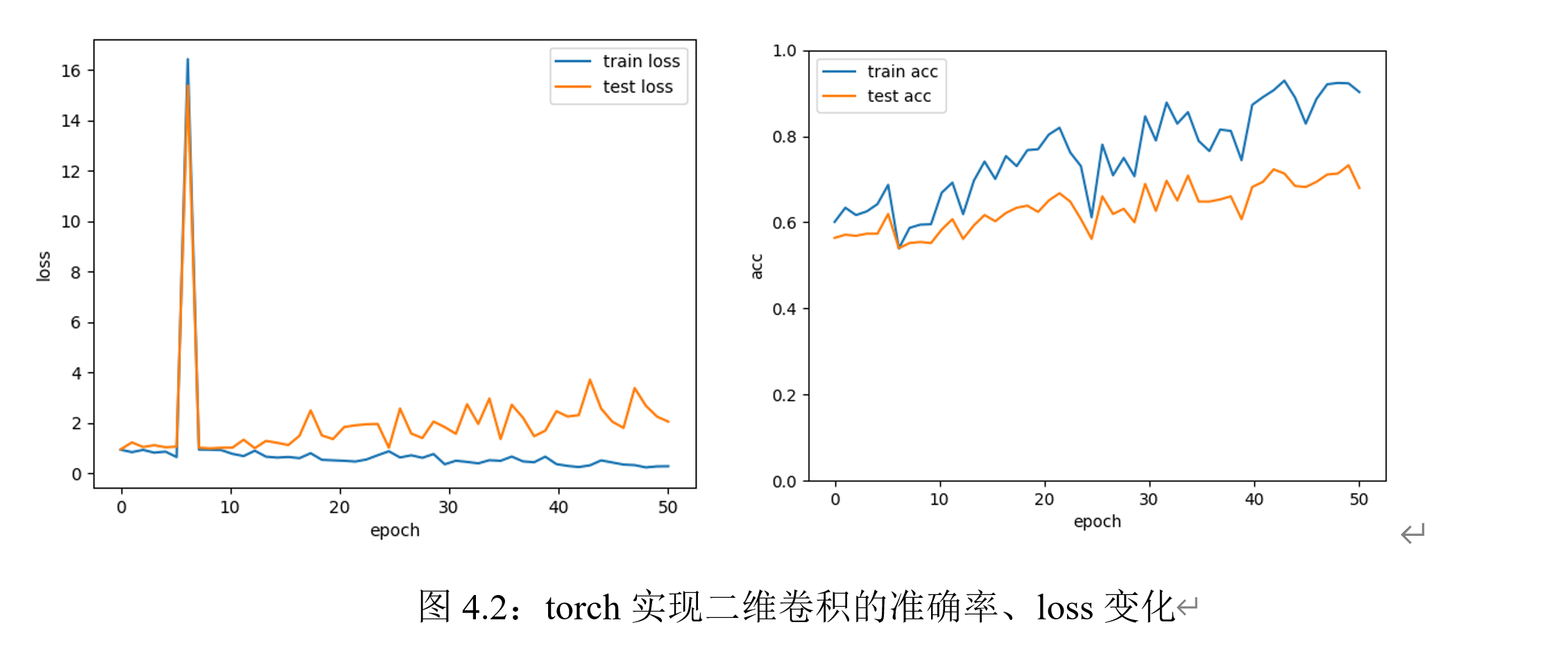实验迭代次数为50次时，在测试集、训练集上的loss和准确率变化如上图所示。可以观察到loss的震荡变化和准确率的逐渐上升；但训练集上的表现始终优于测试集，说明模型存在一定的过拟合现象。在GPU环境下，训练两层卷积神经网络模型训练50次epoch的时间为7分钟左右。
对不同超参数的实验结果对比分析
卷积核大小的影响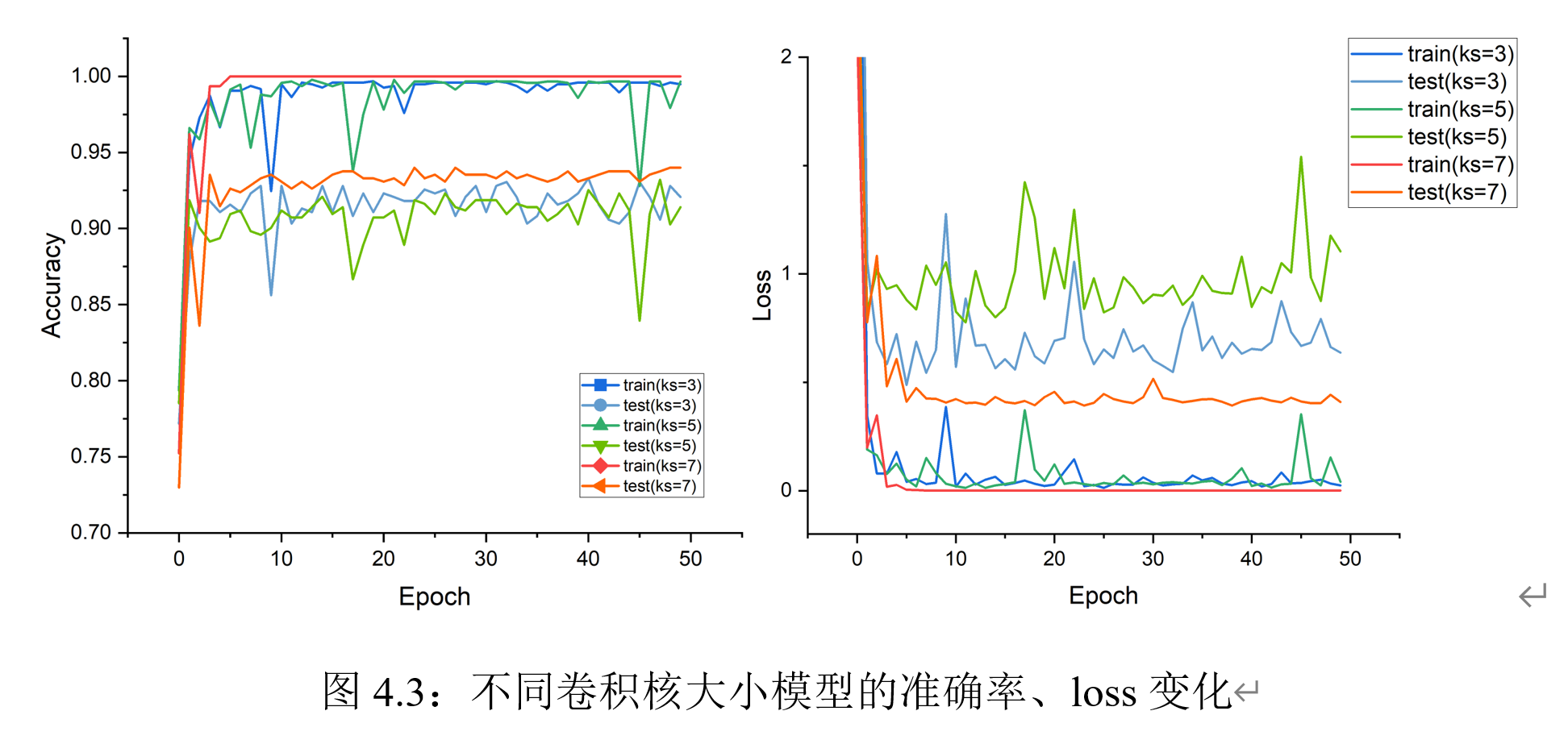可以观察到：尺寸相对较大的卷积核在训练时收敛更快、总体震荡较小，收敛时精度更高、loss更小。尺寸较小的卷积核在训练过程种震荡趋势明显。总体而言，不同尺寸卷积核的模型在此次训练时都存在着过拟合的趋势；可见，是否过拟合和卷积核大小无关。
卷积层数的影响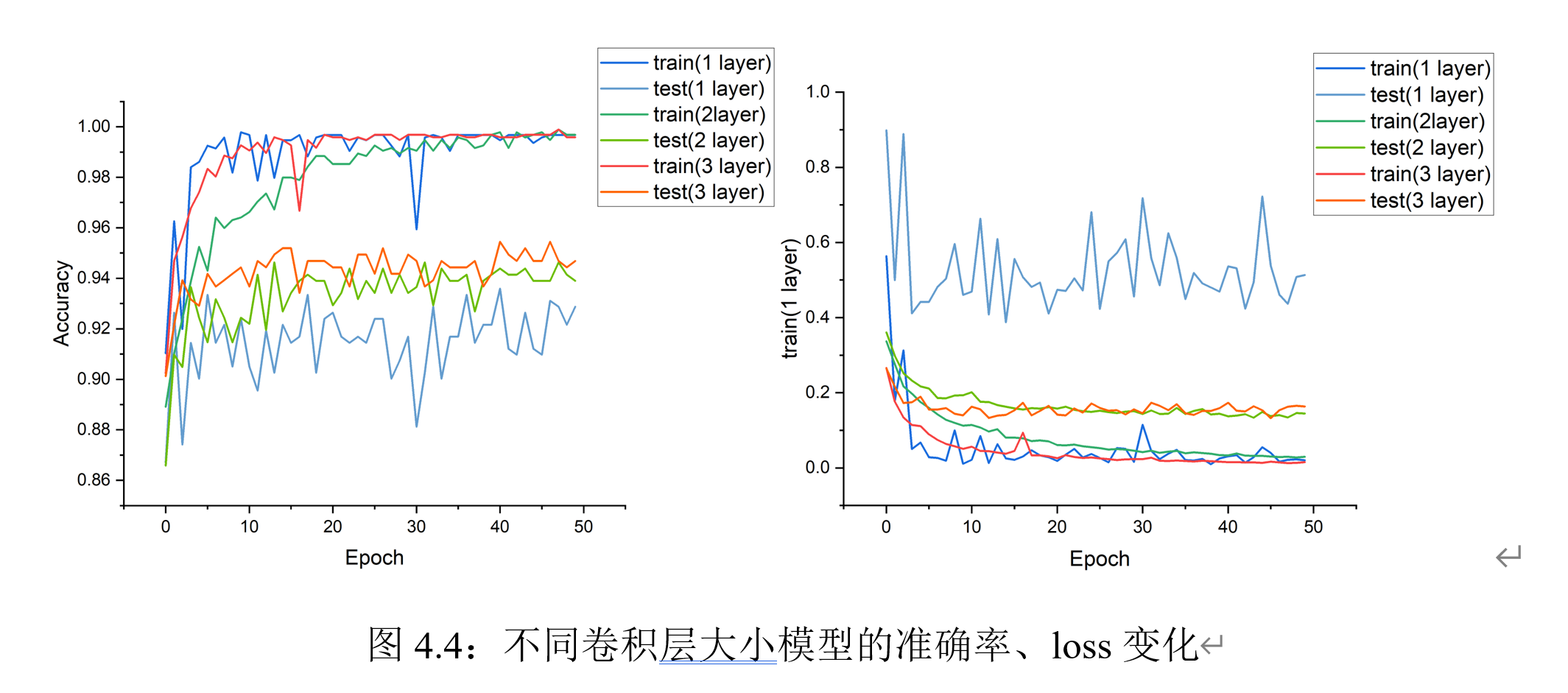可以观察到：随着卷积层数的增加：在训练阶段，训练集上的震荡更小；在收敛阶段，loss更低，在训练集上的准确率更高。在测试集上，三种网络的精确度表现近似，但卷积层数更多的网络相对收敛更快。
复现AlexNet模型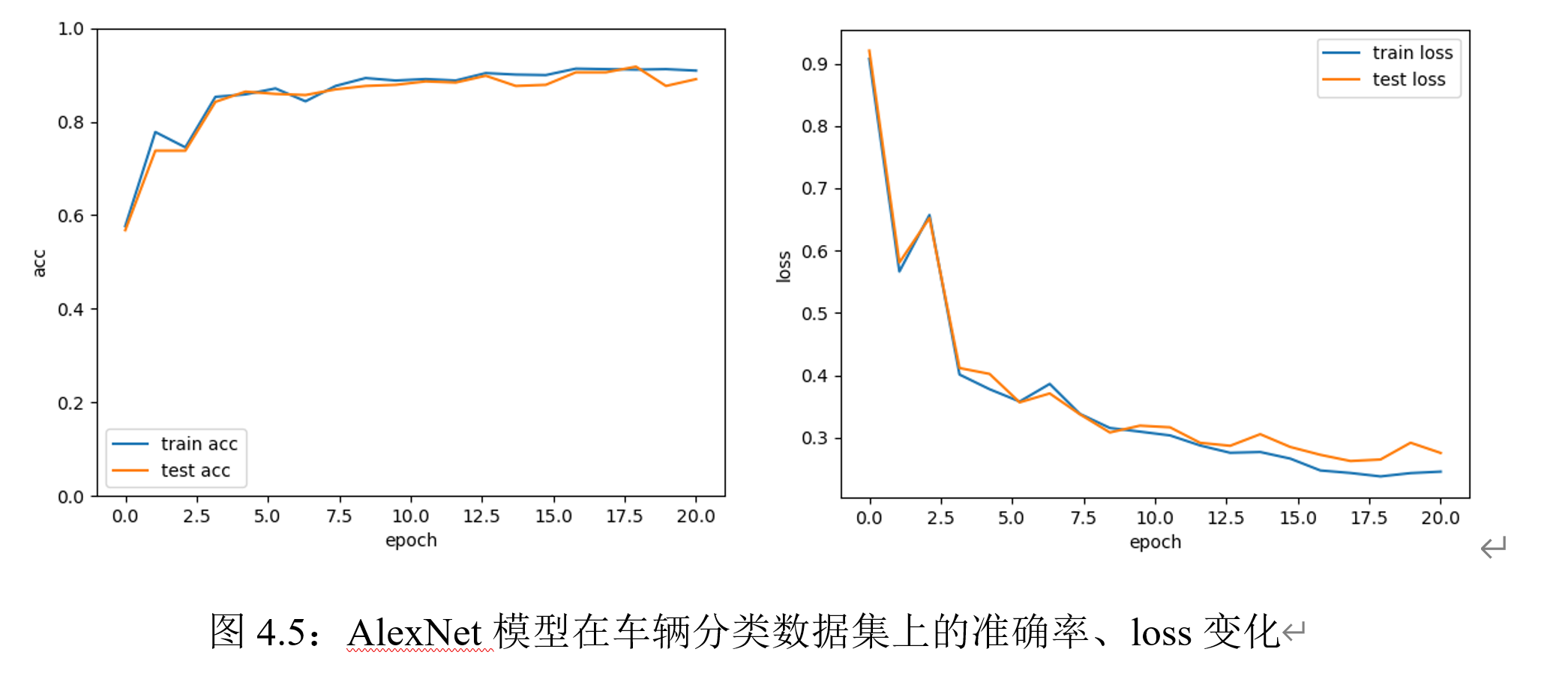在AlexNet上迭代50次的结果如上。可以观察到，随着层数的加深，网络收敛所需的epoch数明显增加。
与前馈神经网络的效果进行对比实验结果表明，在本实验所用的车辆分类数据集上，迭代20次时，前馈神经网络的效果适中，效果最优的是三层卷积神经网络。AlexNet在预测准确度的效果不佳，可能是原因是：（1）数据集过小，模型无法充分学习特征；（2）训练次数不够，模型没有收敛。但值得注意的是，AlexNet在测试集和训练集上的表现都非常接近，说明没有出现过拟合现象。
空洞卷积实验
空洞卷积实验结果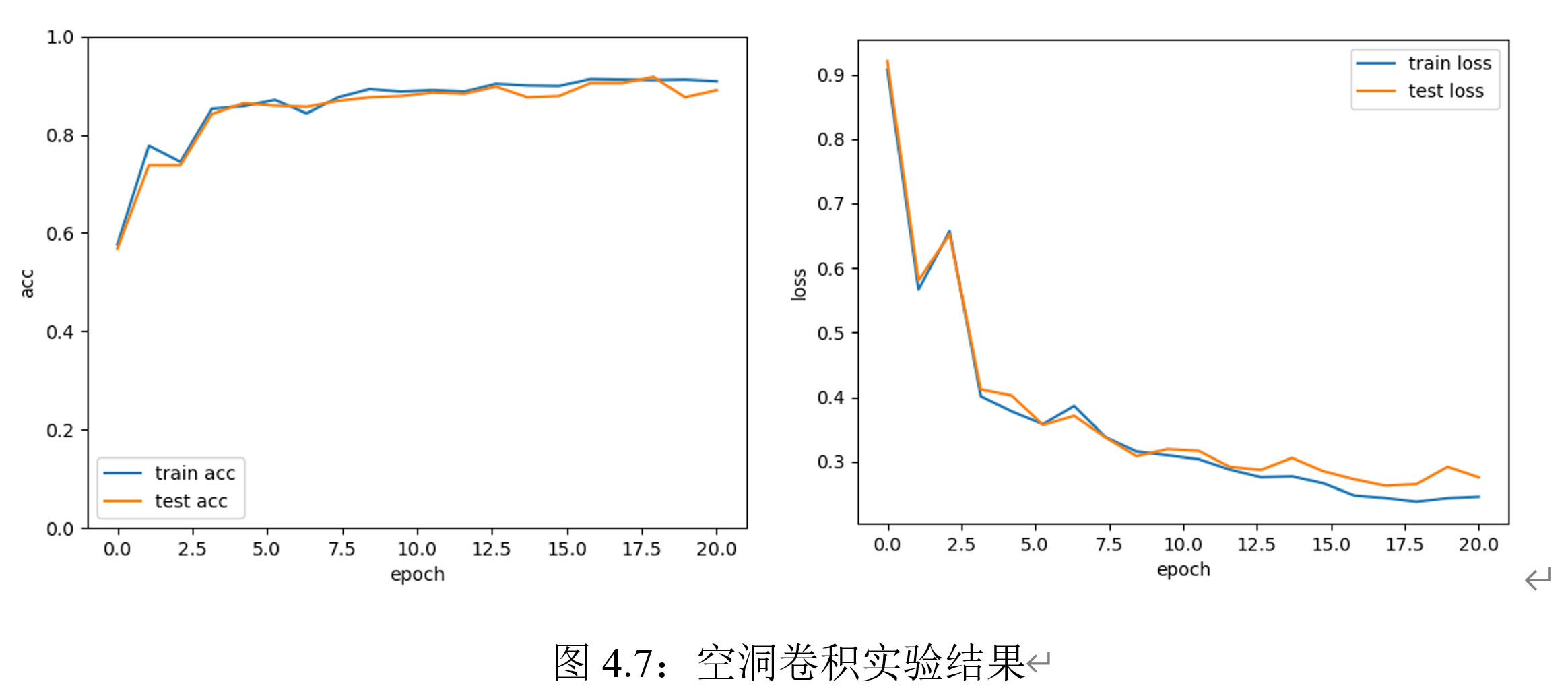空洞卷积的实验结果如上。可以观察到：相比于振荡现象明显的普通卷积，空洞卷积模型没有出现过拟合现象，可能是由于空洞卷积能很好地同时提取远近距离特征。
空洞卷积与普通卷积的效果对比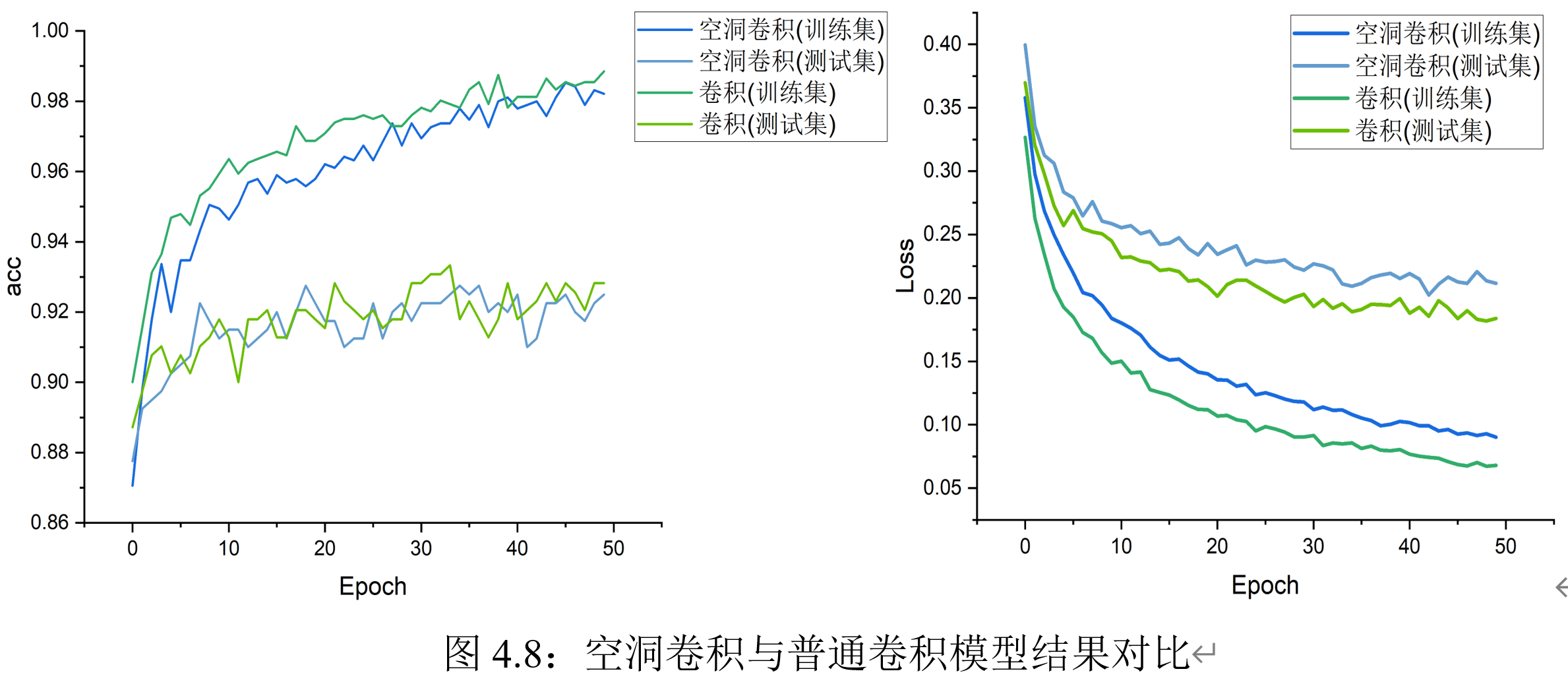从图像可以看出：空洞卷积的准确度变化更平稳、在测试集上的精度和普通卷积类似；在同样训练次数下，空洞卷积模型训练集上的精度略低于普通卷积。可能原因是：空洞卷积提取了更多的层次特征，需要更多的迭代次数才能收敛。 空洞卷积模型相比于普通卷积在时间上略有增加，每次训练平均实际在8分钟左右；但空洞卷积在不同超参数设定下，运行时间变化较大。经过实验验证，可以发现主要耗时的模块在于反向传播部分。
超参数的对比
卷积核大小的影响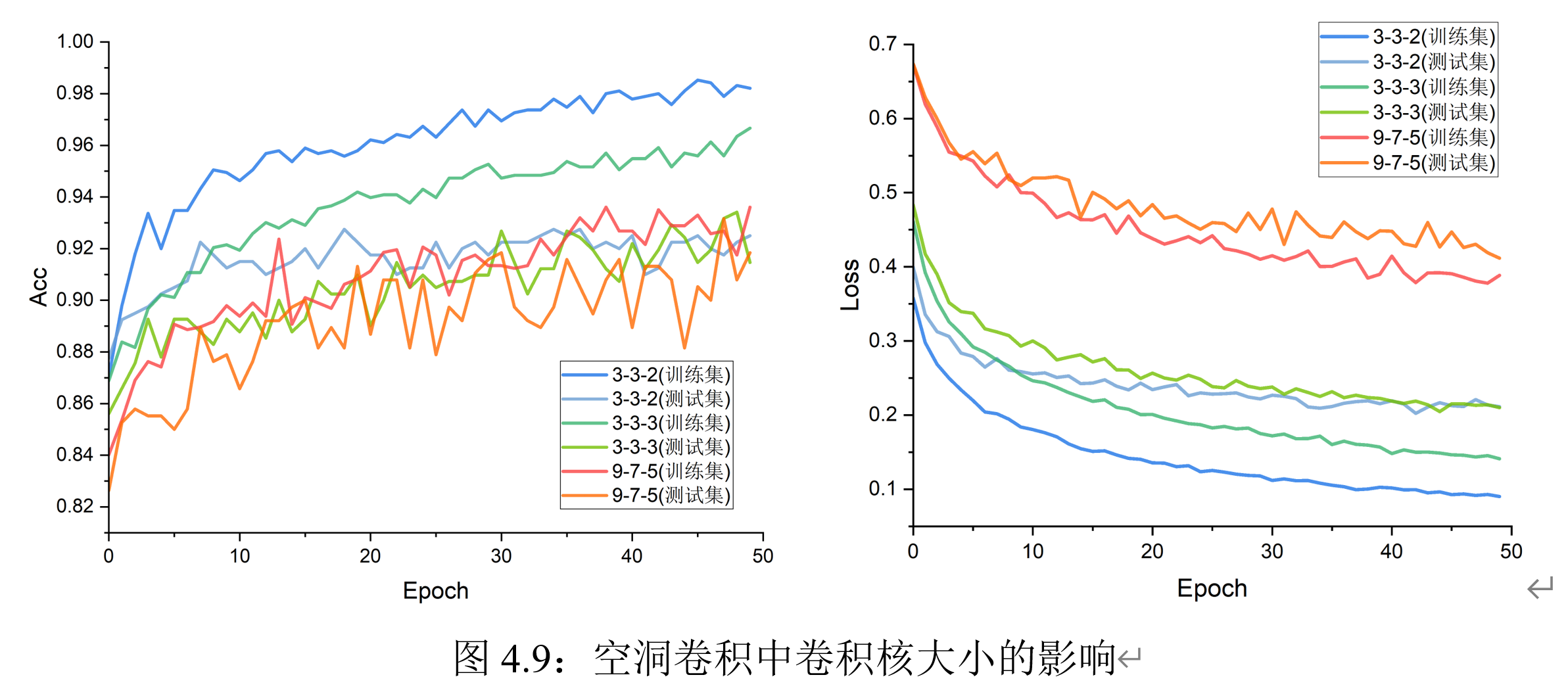实验结果表明：在空洞卷积模型训练前期，卷积核大小分别设置为3、3、2时在测试集上的表现较优、且变化趋势较稳定；在训练后期，模型逐渐收敛，卷积核大小对实验结果的影响减少。可能原因是：卷积核的尺寸增加等价于模型参数量增加，所以在训练前期可能会有更好的表达力。在空洞卷积中，尺寸大的卷积核并没有表现出普通卷积中出现的巨大性能提升。
卷积层数的影响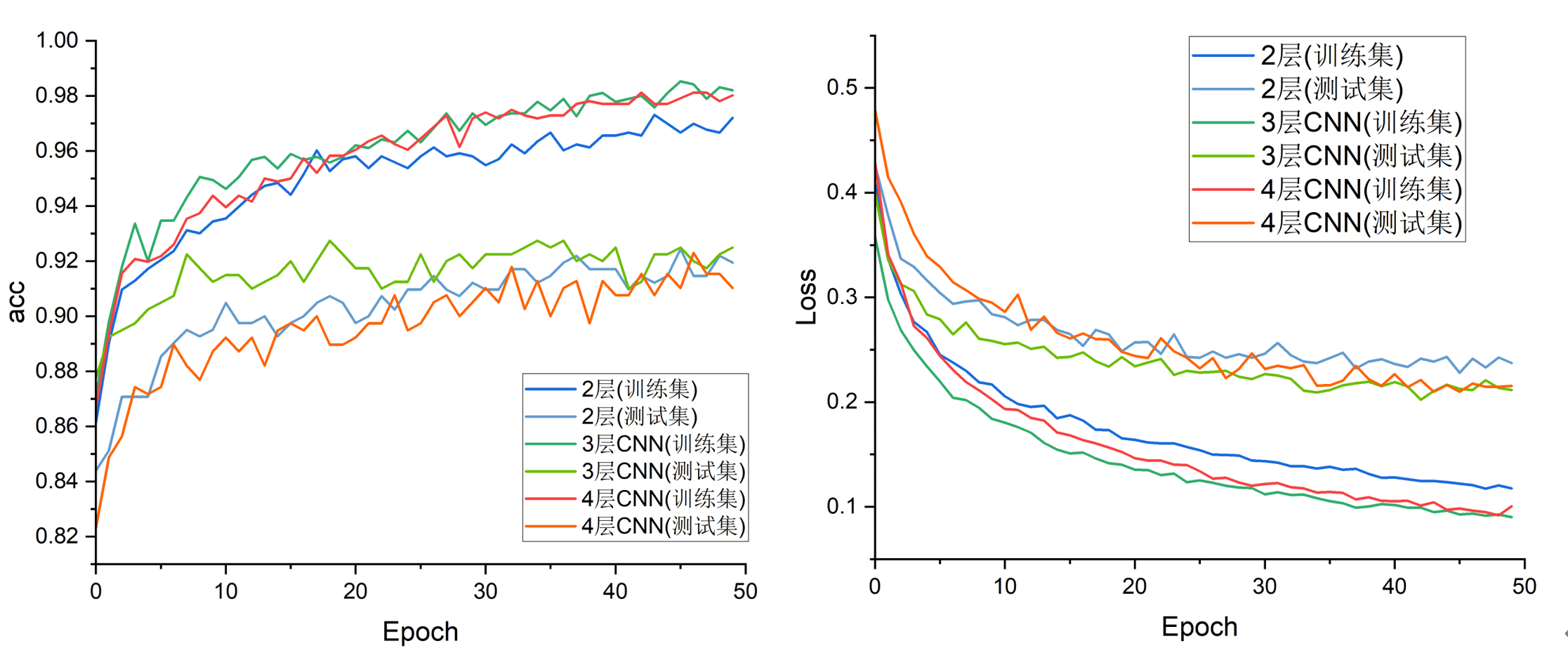可以观察到：在收敛阶段，深层的网络在训练集上准确度和loss上表现更好，但在测试集上没有突出的优势。
残差网络实验
残差网络实验结果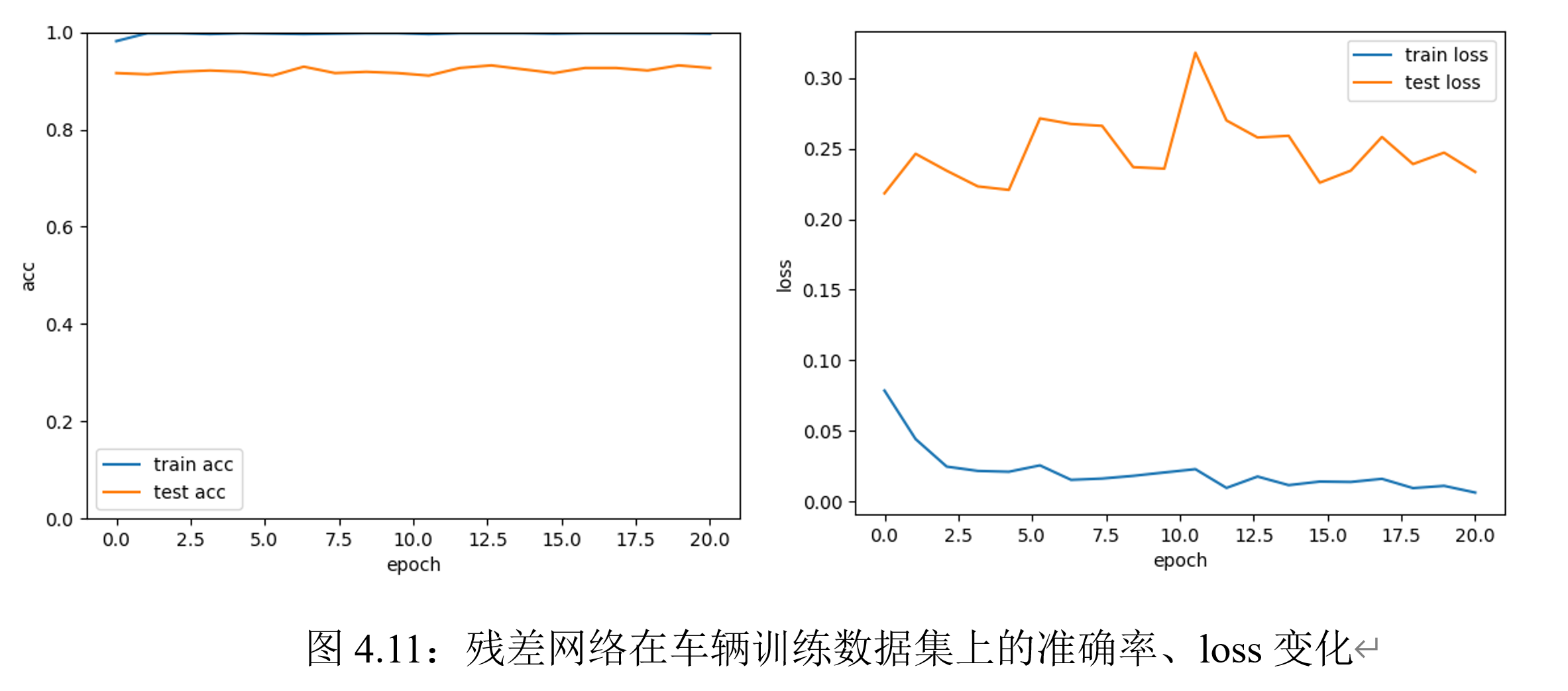观察实验结果可知：残差网络在训练集上迭代20次即可达到接近99%的准确率；此外，模型收敛速度非常快。可见，转化后的函数逼近问题更容易学习。
残差空洞卷积实验结果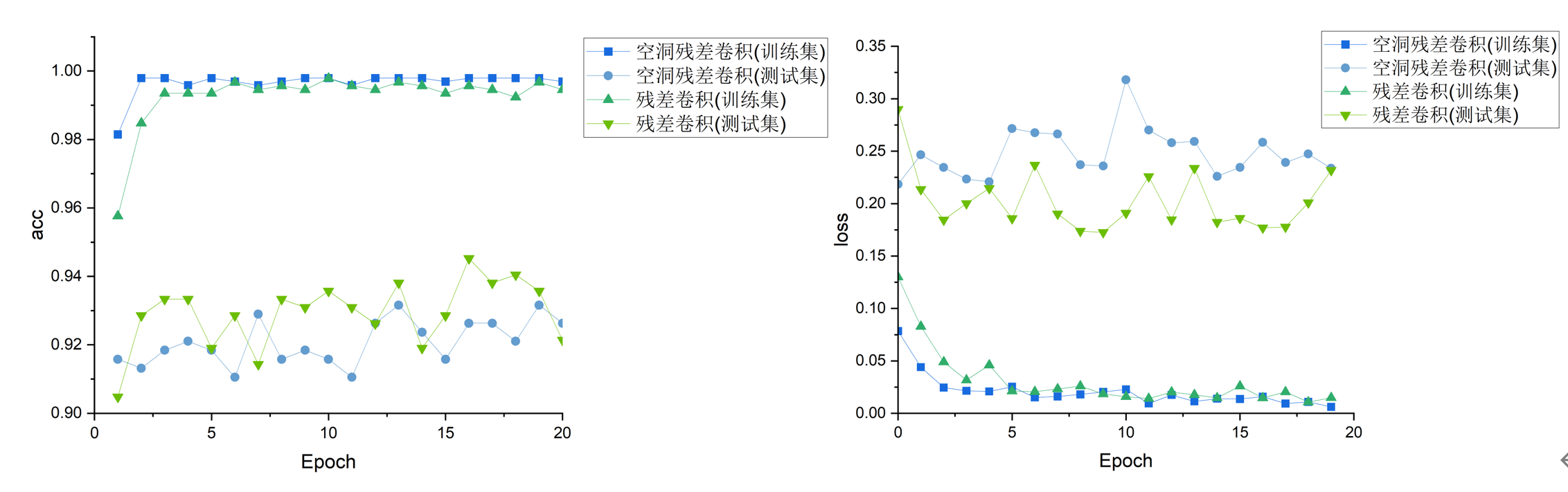实验结果显示，在训练集上，空洞残差卷积具有更高的精度；在测试集上，空洞残差卷积和普通残差卷积表现相似，两者均具有较高的精度。
实验心得体会
本次实验遇到的最大的问题是计算效率的问题；此外，在实验中，还有一些细节可以优化：：
进入卷积神经网络部分后，定义的网络在CPU上的训练时间已远远超出预期；因此，模型迁移到显存、使用GPU进行训练是必须的。在获取服务器使用权限后、进行模型训练后，我直观地体会到GPU带来的效率提升。手动实现的卷积时间复杂度太高，目前仍然无法使用。本实验主要使用torch的实现，没有采用手动卷积。如果后续有可能对此部分进行优化，需要对矩阵乘法部分进行Image to Column的并行化处理。数据集的生成。实验中采用的是OpenCV框架进行读取，但其实OpenCV的通道和RGB颜色的定义和torch中有较大的区别。因此，后续可以考虑使用其它的图片读取框架。超参数的导入。实验中每一层的超参数（卷积层数、卷积核通道数等）是固定的，但这不方便对超参数进行网格搜索。后续可以针对此问题，设置层数的传参。
参考文献
 Pytorch手撕经典网络之AlexNet. https://zhuanlan.zhihu.com/p/29786939  邱锡鹏，神经网络与深度学习，机械工业出版社，https://nndl.github.io/ , 2020.  Krizhevsky A, Sutskever I, Hinton G E. Wang P, Chen P, Yuan Y, et al. Understanding convolution for semantic segmentation[C]//2018 IEEE Winter Conference on Applications of Computer Vision (WACV). IEEE, 2018: 1451-1460.  Zhang, Aston and Lipton, Zachary C. and Li, Mu and Smola, Alexander J. Dive into Deep Learning
写文不易 欢迎投喂！展开全文深度学习 计算机视觉 pytorch 神经网络
• 一：pytorch实现空洞卷积实验（torch实现） 要求： 从至少一个数据集上进行实验，同理，这里我选取了车辆分类数据集（后面的实验都是用的车辆分类数据集），主要在之前利用torch.nn实现二维卷积的基础上，为解决感受...
一：pytorch实现空洞卷积实验（torch实现） 要求： 从至少一个数据集上进行实验，同理，这里我选取了车辆分类数据集（后面的实验都是用的车辆分类数据集），主要在之前利用torch.nn实现二维卷积的基础上，为解决感受野比较的问题，将普通的卷积修改为空洞卷积，并且卷几率符合HDC条件（这里我选取了1,2,5），并且堆叠了2层HDC，即一共六层卷积层。 实验过程： 注：所谓的空洞卷积，与https://blog.csdn.net/qq_37534947/article/details/109726153的torch.nn实现的二维卷积除了模型的定义部分不一样之外，其他的如：数据集、画图、计时、优化器等都是一样的，当然参数设置可能会有些不同，主要在实验结果区分，所以我这里主要针对模型定义做相关介绍。 1.1 空洞卷积模型定义
1.	#pytorch封装卷积层
2.	class ConvModule(nn.Module):
3.	    def __init__(self):
4.	        super(ConvModule,self).__init__()
5.	        #定义六层卷积层
6.	        #两层HDC（1,2,5,1,2,5）
7.	        self.conv = nn.Sequential(
8.	            #第一层 (3-1)*1+1=3 （64-3)/1 + 1 =62
9.	            nn.Conv2d(in_channels = 3,out_channels = 32,kernel_size = 3 , stride = 1,padding=0,dilation=1),
10.	            nn.BatchNorm2d(32),
11.	            # inplace-选择是否进行覆盖运算
12.	            nn.ReLU(inplace=True),
13.	            #第二层 (3-1)*2+1=5 （62-5)/1 + 1 =58
14.	            nn.Conv2d(in_channels = 32,out_channels = 32,kernel_size = 3 , stride = 1,padding=0,dilation=2),
15.	            nn.BatchNorm2d(32),
16.	            # inplace-选择是否进行覆盖运算
17.	            nn.ReLU(inplace=True),
18.	            #第三层 (3-1)*5+1=11  (58-11)/1 +1=48
19.	            nn.Conv2d(in_channels = 32,out_channels = 64,kernel_size = 3 , stride = 1,padding=0,dilation=5),
20.	            nn.BatchNorm2d(64),
21.	            # inplace-选择是否进行覆盖运算
22.	            nn.ReLU(inplace=True),
23.	             #第四层(3-1)*1+1=3 （48-3)/1 + 1 =46
24.	            nn.Conv2d(in_channels = 64,out_channels = 64,kernel_size = 3 , stride = 1,padding=0,dilation=1),
25.	            nn.BatchNorm2d(64),
26.	            # inplace-选择是否进行覆盖运算
27.	            nn.ReLU(inplace=True),
28.	            #第五层 (3-1)*2+1=5 （46-5)/1 + 1 =42
29.	            nn.Conv2d(in_channels = 64,out_channels = 64,kernel_size = 3 , stride = 1,padding=0,dilation=2),
30.	            nn.BatchNorm2d(64),
31.	            # inplace-选择是否进行覆盖运算
32.	            nn.ReLU(inplace=True),
33.	            #第六层 (3-1)*5+1=11  (42-11)/1 +1=32
34.	            nn.Conv2d(in_channels = 64,out_channels = 128,kernel_size = 3 , stride = 1,padding=0,dilation=5),
35.	            nn.BatchNorm2d(128),
36.	            # inplace-选择是否进行覆盖运算
37.	            nn.ReLU(inplace=True)
38.
39.	        )
40.	        #输出层,将通道数变为分类数量
41.	        self.fc = nn.Linear(128,num_classes)
42.
43.	    def forward(self,x):
44.	        #图片经过三层卷积，输出维度变为(batch_size,C_out,H,W)
45.	        out = self.conv(x)
46.	        #使用平均池化层将图片的大小变为1x1,第二个参数为最后输出的长和宽（这里默认相等了）
47.	        out = F.avg_pool2d(out,32)
48.	        #将张量out从shape batchx128x1x1 变为 batch x128
49.	        out = out.squeeze()
50.	        #输入到全连接层将输出的维度变为3
51.	        out = self.fc(out)
52.	        return out


注：可以从上面可以看出一共有6个空洞卷积层，其空洞率分别是1、2、5、1、2、5；1、2、5不包含大于1的公约数，所以其是包含2个的HDC，然后其每一层的输入和输出的算法见注释。
二：pytorch实现残差实验（torch实现） 要求： 从至少一个数据集上进行实验，这里我选取了车辆分类数据集，在给定结构的残差网络中，进行模型的搭建以及训练。 实验结构：实验过程： 这里实验过程和4上面大部分一样，主要是模型设计不同，所以这里主要介绍一下给定的残差网络的模型实现代码。 2.1残差网络块的实现
1.	#残差网络块
2.	#每个残差块都是两层
4.	class ResidualBlock(nn.Module):
5.	    def __init__(self, nin, nout, size, stride=1, shortcut=True):
6.	        super(ResidualBlock, self).__init__()
7.	        #两层卷积层
8.	        #不同步长只有第一层卷积层不同
9.	        self.block1 = nn.Sequential(nn.Conv2d(nin, nout, size, stride, padding=1),
10.	                                    nn.BatchNorm2d(nout),
11.	                                    nn.ReLU(inplace=True),
12.	                                    nn.Conv2d(nout, nout, size, 1, padding=1),
13.	                                    nn.BatchNorm2d(nout))
14.	        self.shortcut = shortcut
15.	        #解决通道数变化以及步长不为1引起的图片大小的变化
16.	        self.block2 = nn.Sequential(nn.Conv2d(nin, nout, size, stride, 1),
17.	                                    nn.BatchNorm2d(nout))
18.	        self.relu = nn.ReLU(inplace=True)
19.
20.	    def forward(self, input):
21.	        x = input
22.	        out = self.block1(x)
23.	        '''''若输入输出维度相等直接相加，不相等改变输入的维度--包括大小和通道'''
24.	        if self.shortcut:
25.	            out = x + out
26.	        else:
27.	            out = out + self.block2(x)
28.	        out = self.relu(out)
29.	        return out


注：这部分主要借鉴的是ppt和网上残差的实现，主要定义了每个残差块内的卷积操作，给定的残差结构内都是两个卷积，，所以这里的self.block1就是其实现，然后因为在残差块的开始会有通道的改变，以及步长的改变，从而导致图片的长和宽变化，所以定义了self.shortcut定义是否会导致变化，然后利用self.block2定义的卷积进行改变输入的大小使得通道可以相加 2.2模型定义
1.	#定义给定的残差结构
2.	class resnet(nn.Module):
3.	    def __init__(self):
4.	        super(resnet, self).__init__()
5.	        self.block = nn.Sequential(nn.Conv2d(3, 64, 3, stride=1, padding=1),
6.	                                   nn.BatchNorm2d(64),
7.	                                   nn.ReLU())
8.	        #t表示2个相同的残差块,每个残差块两个卷积
9.	        self.d1 = self.make_layer(64, 64, 3, stride=1, t=2)
10.	        self.d2 = self.make_layer(64, 128, 3, stride=2, t=2)
11.	        self.d3 = self.make_layer(128, 256, 3, stride=2, t=2)
12.	        self.d4 = self.make_layer(256, 512, 3, stride=2, t=2)
13.
14.	        self.avgp = nn.AvgPool2d(8)
15.	        self.exit = nn.Linear(512, 3)
16.
17.	    def make_layer(self, in1, out1, ksize, stride, t):
18.	        layers = []
19.	        for i in range(0, t):
20.	            if i == 0 and in1 != out1:
21.	                layers.append(ResidualBlock(in1, out1, ksize, stride, None))
22.	            else:
23.	                layers.append(ResidualBlock(out1, out1, ksize, 1, True))
24.	        return nn.Sequential(*layers)
25.
26.	    def forward(self, input):
27.	        x = self.block(input)  # 输出维度 64 * 64 * 64    C * H * W
28.	        x = self.d1(x)  # 输出维度 64 * 54 * 54
29.	        x = self.d2(x)  # i=0 步长为2，输出维度128 * 32 * 32
30.	        x = self.d3(x)  # i=0 步长为2，输出维度256 * 16 * 16
31.	        x = self.d4(x)  # i=0 步长为2，输出维度512 * 8 * 8
32.	        x = self.avgp(x)  # 512 * 1 * 1
33.	        #将张量out从shape batchx512x1x1 变为 batch x512
34.	        x = x.squeeze()
35.	        output = self.exit(x)
36.	        return output


注：这部分就是主要的模型结构的实现，定义了make_layer函数可以进行相同连续残差块的计算，然后调用之前定义好的残差块。
完整代码及数据集下载，见：https://download.csdn.net/download/qq_37534947/13117846
展开全文• 一、空洞卷积的提出 空洞卷积（atrous convolutions）又名扩张卷积（dilated convolutions），向卷积层引入了一个称为 “扩张率(dilation rate)”的新参数，该参数定义了卷积核处理数据时各值的间距。 该结构的...

一、空洞卷积的提出
空洞卷积（atrous convolutions）又名扩张卷积（dilated convolutions），向卷积层引入了一个称为 “扩张率(dilation rate)”的新参数，该参数定义了卷积核处理数据时各值的间距。
该结构的目的是在不用pooling（pooling层会导致信息损失）且计算量相当的情况下，提供更大的感受野。 顺便一提，卷积结构的主要问题如下：

池化层不可学
内部数据结构丢失；空间层级化信息丢失。
小物体信息无法重建 (假设有四个pooling layer 则 任何小于 2^4 = 16 pixel 的物体信息将理论上无法重建。)

而空洞卷积就有内部数据结构的保留和避免使用 down-sampling 这样的特性，优点明显。
二、空洞卷积原理
如下如，卷积核没有红点标记位置为0，红点标记位置同正常卷积核。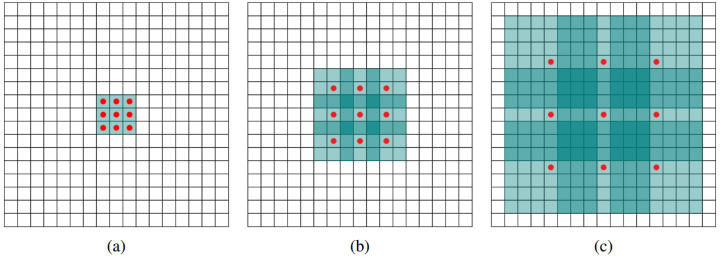假设原始特征为feat0，首先使用扩张率为1的空洞卷积生成feat1，feat1上一点相对feat0感受野为3*3（如图a）；
然后使用扩张率为2的空洞卷积处理feat1生成feat2（如图b），使第一次空洞卷积的卷积核大小等于第二次空洞卷积的一个像素点的感受野，图b即feat1上一个点综合了图a即feat0上3*3区域的信息，则生成的feat2感受野为7*7，即整个图b深色区域；
第三次处理同上，第二次空洞卷积的整个卷积核大小等于第三次空洞卷积的一个像素点的感受野，图c即feat2上每个点综合了feat0上7*7的信息（感受野），则采用扩张率为3的空洞卷积，生成的feat3每一个点感受野为15*15。
相比较之下，使用stride为1的普通3*3卷积，三层之后感受野仅仅为(kernel-1)*layer+1=7。
三、空洞卷积问题
感受野跳跃
我们对同一张图连续三次使用扩张率为1的空洞卷积，观察整张图的中心点的感受野（如下图）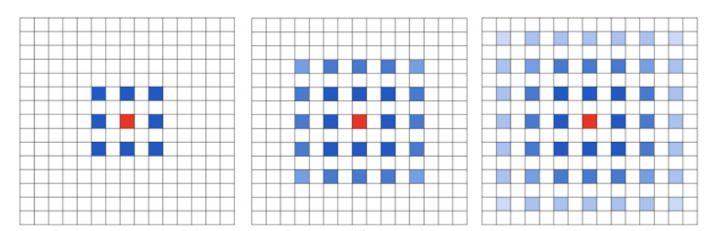很明显，感受野不连续（我们上一小结的例子就没这个问题，所以空洞卷积依赖网络设计）。
小尺度物体检测
类似第一个问题，仍然需要调整扩张率的组合来解决这个问题。
四、网络设计研究

第一个特性是，叠加卷积的 dilation rate 不能有大于1的公约数。比如 [2, 4, 6] 则不是一个好的三层卷积，依然会出现 gridding effect。
第二个特性是，我们将 dilation rate 设计成 锯齿状结构，例如 [1, 2, 5, 1, 2, 5] 循环结构。
第三个特性是，我们需要满足一下这个式子：其中是 i 层的 dilation rate 而是指在 i 层的最大dilation rate，那么假设总共有n层的话，默认。假设我们应用于 kernel 为 k x k 的话，我们的目标则是，这样我们至少可以用 dilation rate 1 即 standard convolution 的方式来覆盖掉所有洞。

一个简单的例子: dilation rate [1, 2, 5] with 3 x 3 kernel (可行的方案)：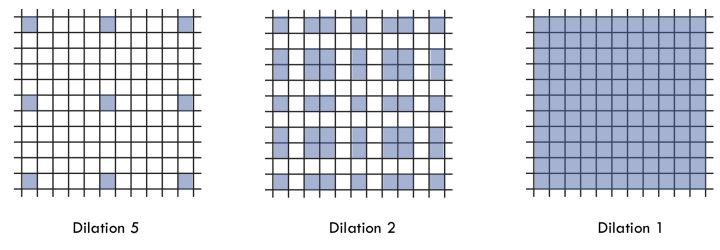而这样的锯齿状本身的性质就比较好的来同时满足小物体大物体的分割要求(小 dilation rate 来关心近距离信息，大 dilation rate 来关心远距离信息)。
单分支设计的研究
通向标准化设计：Hybrid Dilated Convolution (HDC)，可以很好的满足分割需要，如下图所示，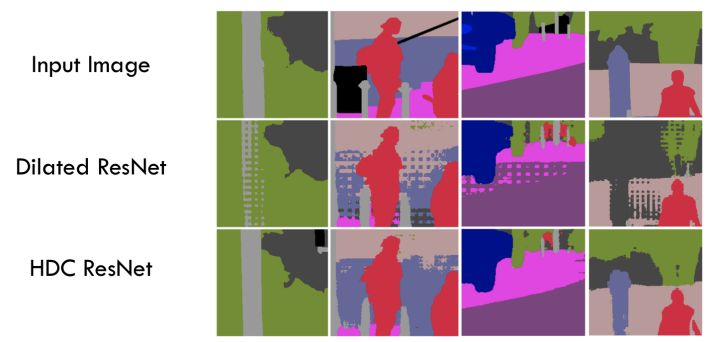多分支研究解决多尺度分割

仅仅(在一个卷积分支网络下)使用 dilated convolution 去抓取多尺度物体是一个不正统的方法。比方说，我们用一个 HDC 的方法来获取一个大（近）车辆的信息，然而对于一个小（远）车辆的信息都不再受用。假设我们再去用小 dilated convolution 的方法重新获取小车辆的信息，则这么做非常的冗余。
基于港中文和商汤组的 PSPNet 里的 Pooling module （其网络同样获得当年的SOTA结果），ASPP 则在网络 decoder 上对于不同尺度上用不同大小的 dilation rate 来抓去多尺度信息，每个尺度则为一个独立的分支，在网络最后把他合并起来再接一个卷积层输出预测 label。这样的设计则有效避免了在 encoder 上冗余的信息的获取，直接关注与物体之间之内的相关性。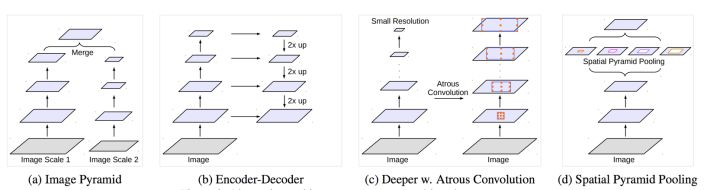五、常用框架API介绍
TensorFlow接口

value： 指需要做卷积的输入图像，要求是一个4维Tensor，具有[batch, height, width, channels]这样的shape，具体含义是[训练时一个batch的图片数量, 图片高度, 图片宽度, 图像通道数]
filters： 相当于CNN中的卷积核，要求是一个4维Tensor，具有[filter_height, filter_width, channels, out_channels]这样的shape，具体含义是[卷积核的高度，卷积核的宽度，图像通道数，卷积核个数]，同理这里第三维channels，就是参数value的第四维
rate： 要求是一个int型的正数，正常的卷积操作应该会有stride（即卷积核的滑动步长），但是空洞卷积是没有stride参数的，这一点尤其要注意。取而代之，它使用了新的rate参数，那么rate参数有什么用呢？它定义为我们在输入图像上卷积时的采样间隔，你可以理解为卷积核当中穿插了（rate-1）数量的“0”，把原来的卷积核插出了很多“洞洞”，这样做卷积时就相当于对原图像的采样间隔变大了。具体怎么插得，可以看后面更加详细的描述。此时我们很容易得出rate=1时，就没有0插入，此时这个函数就变成了普通卷积。

函数默认stride=1，无法改变。
结果返回一个Tensor，填充方式为“VALID”时，返回[batch,height-2*(filter_width-1),width-2*(filter_height-1),out_channels]的Tensor，填充方式为“SAME”时，返回[batch, height, width, out_channels]的Tensor。
测试代码如下：

img = tf.constant(value=[[[,,,],
[,,,],
[,,,],
[,,,]]],dtype=tf.float32)
img = tf.concat(values=[img,img],axis=3)

filter = tf.constant(value=1, shape=[3,3,2,5], dtype=tf.float32)
out_img1 = tf.nn.atrous_conv2d(value=img, filters=filter, rate=1, padding='SAME')
out_img2 = tf.nn.atrous_conv2d(value=img, filters=filter, rate=1, padding='VALID')
out_img3 = tf.nn.atrous_conv2d(value=img, filters=filter, rate=2, padding='SAME')
#error
#out_img4 = tf.nn.atrous_conv2d(value=img, filters=filter, rate=2, padding='VALID')
with tf.Session() as sess:
print('rate=1, SAME mode result:')
print(sess.run(out_img1))
print('rate=1, VALID mode result:')
print(sess.run(out_img2))
print('rate=2, SAME mode result:')
print(sess.run(out_img3)) # error #print 'rate=2, VALID mode result:' #print(sess.run(out_img4))


扩张率为1时，空洞卷积等价于普通卷积。对于SAME和VALID模式计算方式如下图所示，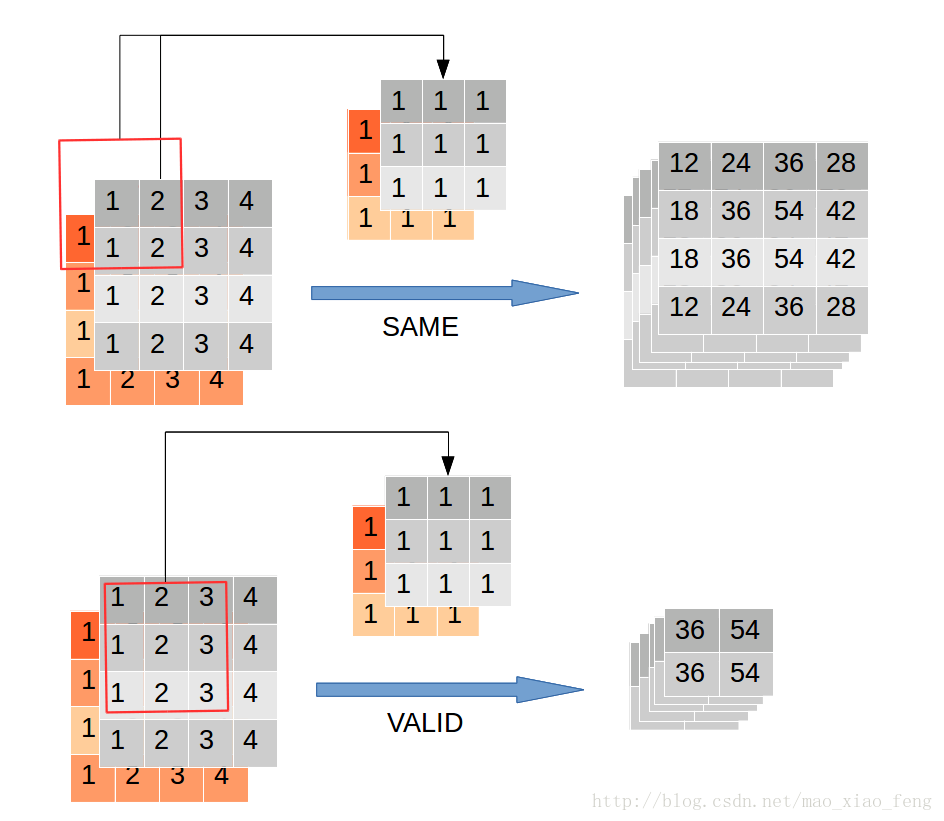扩张率为2的VALID模式计算过程，扩张率为2的VALID模式会报错，此时卷积核大于图片，无法卷积。
MXNet接口
MXNet卷积操作自带扩张率参数，详见文档。
MXNet的通道存储与TensorFlow不太一致，所以我们打印一下（对比上面的图，可以体会到为什么除了tf外大多框架把通道放在第二维），

import  mxnet as mx
import mxnet.ndarray as nd

img = nd.array([[[,,,],
[,,,],
[,,,],
[,,,]]])
img = nd.concat(img, img, dim=-1)
img = nd.transpose(img, axes=(0, 3, 1, 2))

w = nd.ones([5, 2, 3, 3])
b = nd.array([0 for _ in range(5)])
img


[[[[1. 2. 3. 4.]
[1. 2. 3. 4.]
[1. 2. 3. 4.]
[1. 2. 3. 4.]]

[[1. 2. 3. 4.]
[1. 2. 3. 4.]
[1. 2. 3. 4.]
[1. 2. 3. 4.]]]]
<NDArray 1x2x4x4 @cpu(0)>

nd.Convolution(img, w, b, kernel=w.shape[2:], num_filter=w.shape, stride=(1, 1), pad=(1,1), dilate=(1,1))


[[[[12. 24. 36. 28.]
[18. 36. 54. 42.]
[18. 36. 54. 42.]
[12. 24. 36. 28.]]

[[12. 24. 36. 28.]
[18. 36. 54. 42.]
[18. 36. 54. 42.]
[12. 24. 36. 28.]]

[[12. 24. 36. 28.]
[18. 36. 54. 42.]
[18. 36. 54. 42.]
[12. 24. 36. 28.]]

[[12. 24. 36. 28.]
[18. 36. 54. 42.]
[18. 36. 54. 42.]
[12. 24. 36. 28.]]

[[12. 24. 36. 28.]
[18. 36. 54. 42.]
[18. 36. 54. 42.]
[12. 24. 36. 28.]]]]
<NDArray 1x5x4x4 @cpu(0)>

nd.Convolution(img, w, b, kernel=w.shape[2:], num_filter=w.shape, stride=(1, 1), pad=(2,2), dilate=(2,2))


[[[[16. 24. 16. 24.]
[16. 24. 16. 24.]
[16. 24. 16. 24.]
[16. 24. 16. 24.]]

[[16. 24. 16. 24.]
[16. 24. 16. 24.]
[16. 24. 16. 24.]
[16. 24. 16. 24.]]

[[16. 24. 16. 24.]
[16. 24. 16. 24.]
[16. 24. 16. 24.]
[16. 24. 16. 24.]]

[[16. 24. 16. 24.]
[16. 24. 16. 24.]
[16. 24. 16. 24.]
[16. 24. 16. 24.]]

[[16. 24. 16. 24.]
[16. 24. 16. 24.]
[16. 24. 16. 24.]
[16. 24. 16. 24.]]]]
<NDArray 1x5x4x4 @cpu(0)>

六、参考来源
Multi-scale Context Aggregation by Dilated Convolutions
【Tensorflow】tf.nn.atrous_conv2d如何实现空洞卷积？
如何理解空洞卷积（dilated convolution）？


展开全文• 空洞卷积能够在不减小感受野的情况下降低空间特征的损失，可以获取long-ranged information。但是也有弊端：比如空间上的连续所能给出的信息可能因此而丢失(比如边缘之类的)，同时对于小物体的分割未必有利。同时，...
• 针对高光谱影像分类时光谱维数据量巨大的特点,提出一种基于三维空洞卷积残差神经网络的高光谱影像分类方法。该方法以高光谱像元立方体作为数据输入,使用三维卷积核同时提取高光谱数据的空间维和光谱维特征,并通过在...
• 其中，rf为当前层的每个神经元的感受野大小，k为真实的卷积核大小（与dilation系数有关），rf_stride为当前层的感受野步长，其为前馈网络父节点所有stride的连乘。则由rf = rf + rf_stride * (k-1)可计算出当前层的...
每个卷积层可视为三元组[kernel_size, stride, dilation]， 每个最大池化层可视为二元组[1, stride]。 以一个类似Deeplabv3+的Resnet50为例。 总共有4个block，每个block的layer个数为：[3, 4, 6, 3]。 以最后一个block的参数为例，有[[3, 1, 1], [3, 1, 2], [3, 1, 4]]。 感受野计算代码如下：
layers = [[7, 2], [1, 2], [3, 1], [3, 1], [3, 1], [3, 2], [3, 1], [3, 1], [3, 1], [3, 2],[3, 1], [3, 1], [3, 1], [3, 1], [3, 1], [3, 1, 1], [3, 1, 2], [3, 1, 4]]
rf_stride = 1
rf = 1
for layer in layers:
if len(layer) == 2:
k = layer
stride = layer
if len(layer) == 3:
kernel_size = layer
stride = layer
dilation = layer
k = (dilation-1)*(kernel_size-1)+kernel_size
rf = rf + rf_stride * (k-1)
rf_stride *= stride
print(rf)

其中，rf为当前层的每个神经元的感受野大小，k为真实的卷积核大小（与dilation系数有关），rf_stride为当前层的感受野步长，其为前馈网络父节点所有stride的连乘。则由rf = rf + rf_stride * (k-1)可计算出当前层的输出的感受野大小。
展开全文感受野 卷积神经网络 深度学习 机器视觉
• [卷积]空洞卷积的改进 文章来源： https://zhuanlan.zhihu.com/p/50369448 从这几年的分割结果来看，基于空洞卷积的分割方法效果要好一些，为此，拿出两天时间来重新思考下空洞卷积问题。- . -语义...
• ## 空洞卷积

千次阅读 2018-10-23 19:55:04
Dilated/Atrous Convolution 或者是 Convolution with holes 从字面上就很好理解，是在标准的 convolution map 里注入空洞，以此来增加 reception field。相比原来的正常convolution，dilated convolution ...
• 空洞卷积：在3*3卷积核中间填充0，有两种实现方式，第一，卷积核填充0，第二，输入等间隔采样。 空洞卷积有什么作用呢？ 扩大感受野 在deep net中为了增加感受野且降低计算量，总要进行降采样(pooling或s2/conv)，...算法 python 深度学习 计算机视觉
• CNN从2012年的AlexNet发展至今，科学家们发明出各种各样的CNN模型，一个比一个深，一个比一个准确，一个比一个轻量。我下面会对近几年一些具有变革性的工作进行简单盘点，...Group convolution 分组卷积，最早在AlexN.神经网络 网络 机器学习 计算机视觉
• 卷积核：分组卷积、可分离卷积、空洞卷积、可变形卷积 CNN从2012年的AlexNet发展至今，科学家们发明出各种各样的CNN模型，一个比一个深，一个比一个准确，一个比一个轻量。我下面会对近几年一些具有变革性的工作进行...
• 然而，空洞卷积也会造成网格伪影的现象，这严重阻碍了在DCNN中应用了空洞卷积的表现。在本工作中，作者提出了两种简单但有效的去网格方法，是通过研究分解空洞卷积实现来完成的。该工作不像目前已经存在的方法，那些...深度学习 python
• 文章目录分组卷积（Group Convolution）原理用途常规卷积（Convolution）空洞（扩张）卷积（Dilated/Atrous Convolution）深度可分离卷积（depthwise separable convolution）标准卷积与深度可分离卷积的不同深度可...
• 我大概描述一下这个网络的结构： 首先输入数据，这里我们输入的是音频的mfcc特征（不懂没关系，等会说）， 接着进行一个一维的空洞卷积，然后进入到残差块中，残差块是这样的结构：将进来的数据再进行一次空洞卷积...语音识别 WAVENet keras 自然语言处理
• 空洞卷积 deeplabby Thalles Silva 由Thalles Silva 深入探讨深度卷积语义分割网络和Deeplab_V3 (Diving into Deep Convolutional Semantic Segmentation Networks and Deeplab_V3) Deep Convolutional Neural ...网络 算法 python 计算机视觉 神经网络
• 目标检测新网络 DetectoRS：54.7 AP，特征金字塔与空洞卷积的完美结合 论文地址：https://arxiv.org/abs/2006.02334 代码地址（基于mmdetection实现）： https://github.com/joe-siyuan-qiao/DetectoRS 本文是谷歌...
• 文章转自：同作者个人微信公众号【机器学习炼丹术】。欢迎交流沟通，共同进步，作者微信：cyx...膨胀卷积，又叫做空洞卷积，是在卷积核参数不变的基础上，增加视野域的一种手段。通过使用膨胀卷积，可以放大最深层特机器学习 人工智能 计算机视觉 网络
• ## 第五章 卷积神经网络

千次阅读 多人点赞 2020-01-13 07:49:37
第五章 卷积神经网络第五章 卷积神经网络卷积一维卷积二维卷积互相关卷积的变种卷积的数学性质交换性导数卷积神经网络用卷积来代替全连接卷积层汇聚...-5AlexNetInception 网络残差网络其它卷积方式转置卷积空洞卷积...
• 因此文章提出使用空洞卷积作为分类网络的卷积，并通过实验证明了空洞残差网络的性能比普通残差网络的性能超出很多。同时文章也提出了类似 Understanding Convolution for Semantic Segmentation 中提出的 g r i d d ...膨胀
• 卷积神经网络进阶用法---变形卷积核？分离卷积核？。。。 我在三个月前写了关于卷积神经网络的系列文章，很短时间内就有了上千阅读量，深感荣幸。说明当前读者对深度学习的关注度是相当高的，之前的系列文章主要是......